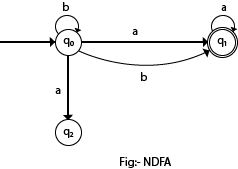# Non Deterministic Finite Automata | Language of NFA | Extended transition function of NFA | Theory Of Computation (TOC)## Non Deterministic Finite Automata (NFA):

Like the DFA, a NFA has a ﬁnite set of states, a finite set of input symbols, one start state, a set of accepting states and transition function (𝛿). The difference between the DFA and the NFA is in the type of 𝛿. For the NFA, 𝛿 is a function that takes a state and input symbol as arguments as like the DFA’s transition function, but returns a set of zero, one or more state (DFA returns exactly one state).

#### Formal Deﬁnition

A non deterministic ﬁnite automaton is a quintuple A: (Q, ∑, 𝛿, qn, F), where
Q = ﬁnite set of states.
∑= ﬁnite set of input symbols.
𝛿= transition function that maps Q✕∑→2^ Q
qo = a member of Q, is the start state.
F = a subset of Q, is the set of ﬁnal states.

Adding the non-determinism to ﬁnite state machine doesn’t increase the power of computability, but it makes ﬂexible to construct finite state machine to represent the language. So. the computation power of DFA and NFA is not different, only to construct a NFA is easier than DFA.

For example:

Fig: NFA accepting all strings that end on 01

Here, from state q, there is no any arc for input symbol 0 & no any arc out of Q3 for 0 & l. So, we can conclude in a NFA, there may be zero no. of arcs out of each state for each input symbol.While in DFA, it has exactly one arc out of each state for each input symbol.

Fig: Transition table for NFA accepting all strings that end on 01

• NFA over {0, 1} accepting strings {0, 01, 11}.

Computation tree for 01 10;

The computation tree of the processing of any string by an NFA represents the entire path from start state that can follow by an NFA for processing that string. The root of tree is the start state. After completing the tree for a string of length ‘n’, if there is any ﬁnal state node at level ‘n’, then the NFA accepts that string otherwise doesn’t.
In above example,
|01|= 2
At level 2, there is 21 final state node (q2). So, 01 is accepted by a NFA.

### The Extended transition function of NFA:

As for DFA’s, we need to deﬁne the extended transition function 𝛿 that takes a state q and a string of input symbol w and returns the set of states that is in if it starts in state q and processes the string w.

Definition by Induction:
Basis Step: Then, 𝛿cap (q, a) = {q} i.e. reading no input symbol remains into the same state.
Induction: Let w be a string from ∑* such that w = xa, where x is a substring of without last symbol a. Also, Suppose that,
𝛿cap(q, x) = {p1,p2,p3,....,pt}
Let,

Then, 𝛿cap(q, w) = {r1,r2,  ....,rm}
We compute  𝛿cap(q, w) by ﬁrst computing 𝛿cap(q, x) and then following any transition from any of these states that is labeled a.

Let us consider a NFA accepting all strings that end on 01:

Here, we use 𝛿cap to describe how the string 00101 is processed by the NFA.

### Language of NFA:

The language of NFA, A = (Q, ∑,𝛿, qo, F), denoted by L (A):
L(A)= {w|𝛿cap(q,w)  F≠φ}
i.e. L(A) is the set of strings W in ∑* such that 𝛿cap(qo,w) contains at least one state accepting state.